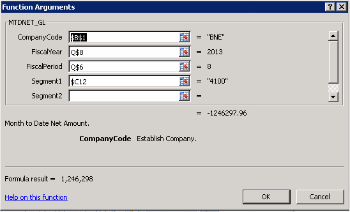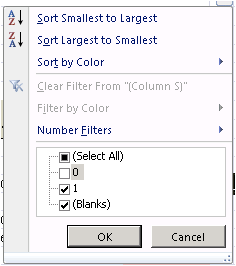Are you still clinging to FRx because you simply don’t have the time or willpower to learn a new financial reporting software? Do you know Excel? If so, it’s time to move to BizInsight by BizNet Software!

Tune in to one of our webinars for an introduction and brief demo of BizInsight in action, or contact Jody Patterson ( jody@oasisky.com) for more information.

For those who have already embraced BizInsight, here’s one of the Top 10 BizNet Tips and Tricks:

Rolling 12 Month IS with Zero Suppression

Using Excel and BizInsight logic, you can turn a static 12 month Income Statement into a rolling 12 month Income Statement. With a rolling 12 month Income Statement, you can update an entire report in a single cell.

1. Set cell Q6 = B3 (the current period you will change in your parameter box)
2. For the other periods, starting in cell P6, use the formula =IF(Q\$6-1=0,12,Q\$6-1)
• This formula means if the current period minus 1 is 0, then change the cell to period 12, otherwise take the current period minus 1
3. Set Q8 = B2 (the current year in your parameter box)
4. For the other years, starting in cell P8, use the formula =IF(P\$6=12,Q\$8-1,Q\$8)
• This formula means if the period is 12, than do the current year minus 1, otherwise take the current year
5. Make sure the year and period in the function argument box point to the year and period in the same column.  For example, all the functions in column Q would have the following year and period
• NOTE: Excel Anchoring is very important in this report6. Use the formula =IF(AND(MIN(F12:R12)=0,MAX(F12:R12)=0),0,1) to suppress the rows that have only zero amounts
• Fill in the range for the MIN and MAX for the rows that you want the formula to check for zero rows.  In this example, columns F:R is the range that should be input in the above formula
• Best Practice: Hard code a 1 in the rows that you do not want suppressed.
• Hardcoding a 1 in rows that you do not want suppressed will ensure that these rows will never be hidden while using the zero row suppression filter
7. Apply an Excel filter to the column you put your zero row suppression formula in.  In this example, the filter is in column S.
8. Uncheck the 0 and those rows will be suppressed9. Change the font color in column S to white. This will allow the column to remain hidden for report design but will keep the column visible to reapply the filter.

Scroll To Top